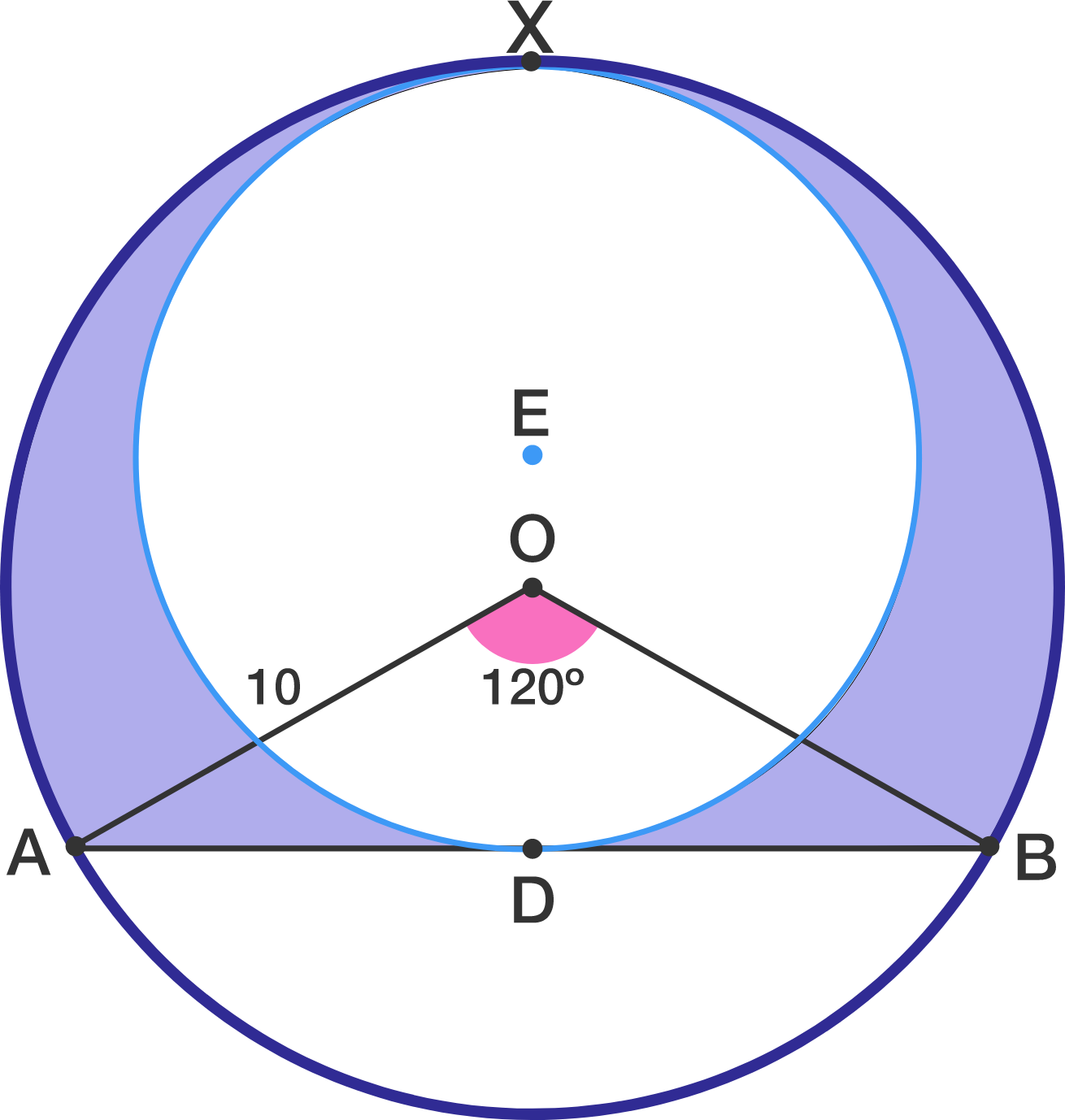# Tempting Circle

Geometry Level 2In a circle with center $O$, a chord $AB$ is drawn such that $\angle AOB = 120^\circ$. Radius $AO=10$. A circle is drawn in the major arc such that it's radius is maximum, with center E, it touches the larger circle at point X as shown in figure.

The area of the shaded region can be expressed as $\dfrac{a\pi +b\sqrt{3}}{c}$ for integers $a,b,c$ with $\gcd(a,c)=1$ What is the value of $a+b+c$?

×

Problem Loading...

Note Loading...

Set Loading...